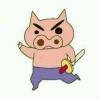{meta: 原调: 1=D} {meta: 选调: 1=C} {meta: Capo=2} {comment: 6323 5323 4323} {start_of_tab} {end_of_tab} {comment: 主歌 A} [E] 我在[G]阴暗中降[D]落 [E] 世界[A]在雨中淹[Em]没 [E] 画面[G]与现实交[A]错 无[D]法抽离卡在胸[Em]口 [E] 躯壳[G]如行尸走[D]肉 [E] 陷阱[A]旋涡我已受[E]够 [E] 挣脱[G]逃离这个[A]空洞 [E] 如[E]果我冲出黑幕笼罩的天空 就别想在捆绑我的自[E]由 在[E]狂风之中[G] 嘶[G]吼 作[D]困兽之斗[Em] 我[E]奋力冲破[G] 封[D]闭的思绪[Em]震开裂缝 燃[E]烧的花朵[G] 升[G]空 消[D]失在空中[Em] 记[E]忆在剥落[G] 残[D]留的影像[E]轮廓 [E] 溃散在[A]薄雾[E]中 (2)[Em] 如果 [Em] 我冲出黑幕笼罩[G]的天空[Em] 就[D]别在捆绑[A]我的自由 {end_of_chorus} {title:困兽之斗} {artist:周杰伦} {author:鑫哥}电脑科技